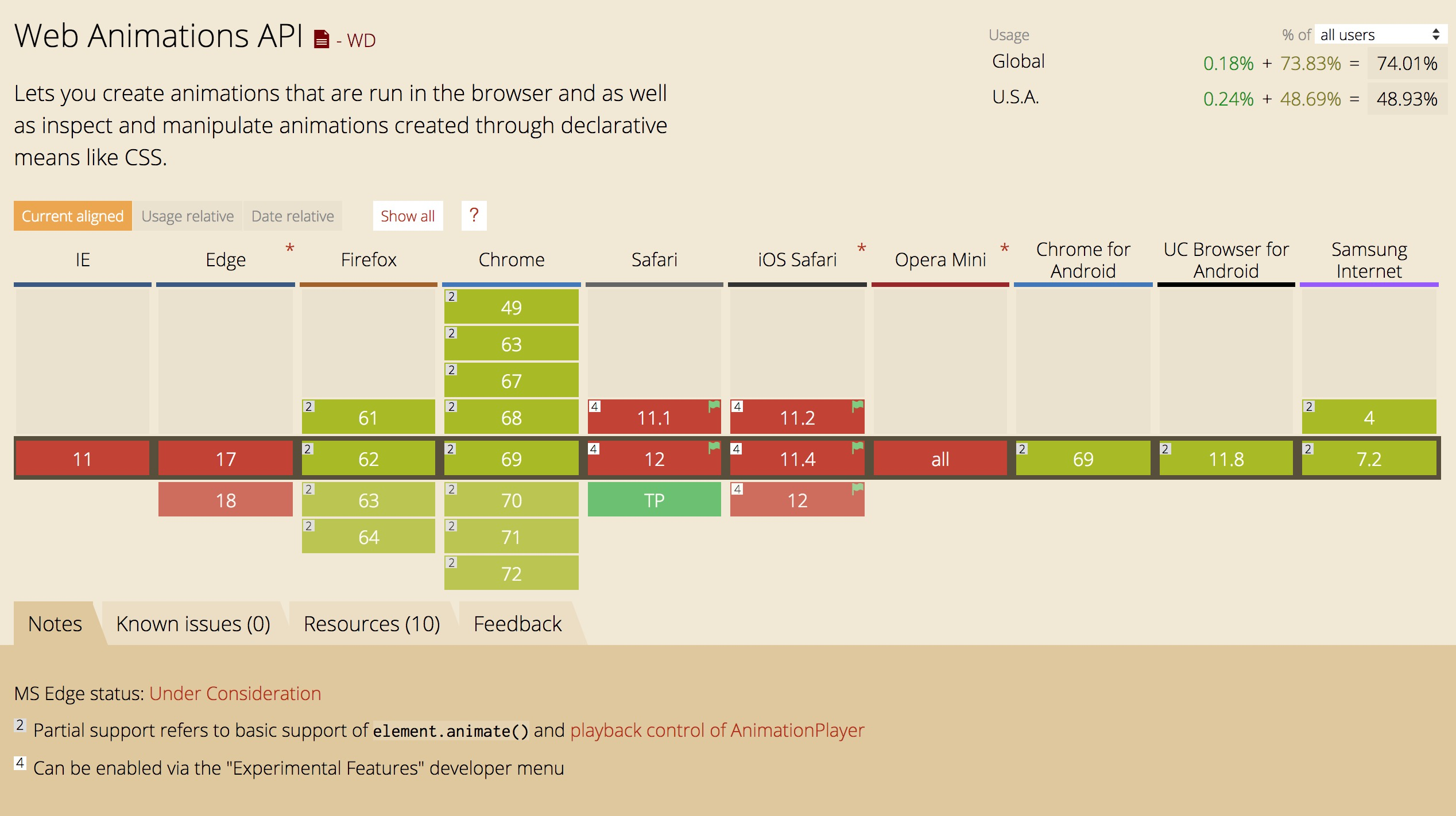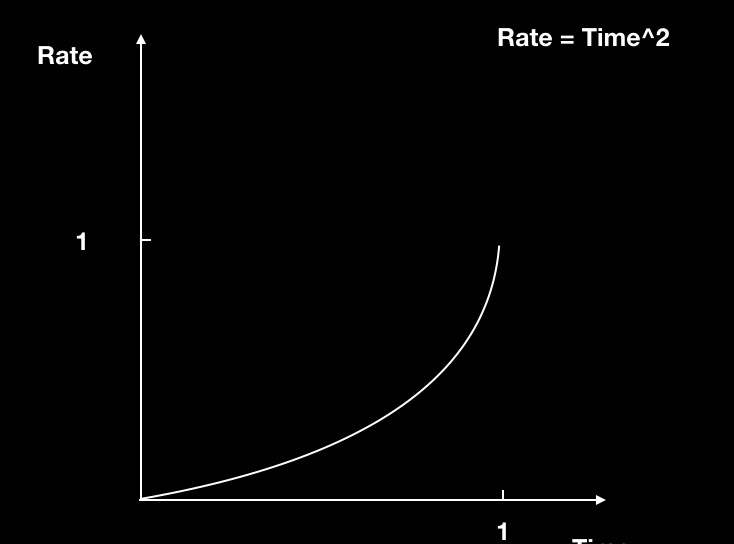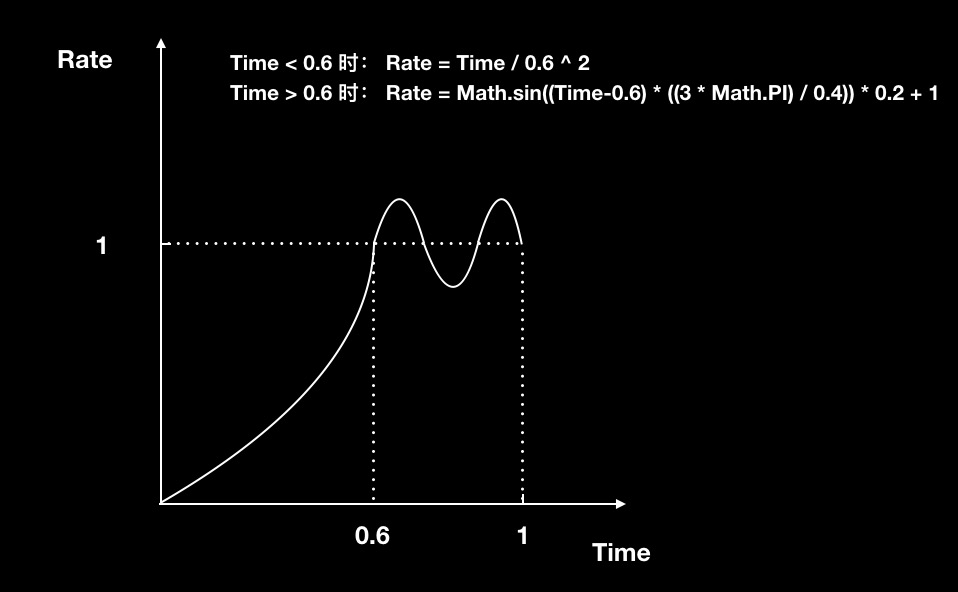# 大话 JavaScript 动画

## 背景

138.2亿年前，世界上没有时间和空间，或许世界都不存在，在一个似有似无的点上，汇集了所有的物质，它孕育着无限的能量与可能性。

## 宇宙大爆炸## 人类起源## 动画的形成## 前端动画实现

Atwood 定律：Any application that can be written in JavaScript will eventually be written in JavaScript.

### DOM动画中的 CSS3

CSS3 动画是当今盛行的 Web 端制作动画的方式之一，对于移动设备来说覆盖率已经非常广泛，在日常开发中可以使用。CSS3 动画只能通过对 CSS 样式的改变控制 DOM 进行动画

### DOM动画中的 WebAnimation

WebAnimation 还在草案阶段，在Chrome可以尝试使用一下。移动设备还是相当惨烈，iOS 并没有开始支持。

Element Animation MDNCSS3 和 WebAnimation 都只能作用于DOM，那么，如果我们想让 Canvas 上的对象产生动画，那我们该怎么办呢？

### JavaScript

#### 元素移动

``````<div class=“box”></div>
``````

``````.box {
width: 100px;
height: 100px;
background: red;
}
``````
``````const box = document.querySelector('.box') // 获取方块元素
let value = 0 // 设置初始值
// 创建每一帧渲染之前要执行的方法
const add = () => {
value += 5 // 每帧加数值增加5
box.style.transform = `translateX(\${value}px)` // 将数值设置给 方块 的 css 属性 transform 属性可以控制元素在水平方向上的位移
}
``````

#### 补间动画

``````/**
*  执行补间动画方法
*
* @param      {Number}    start     开始数值
* @param      {Number}    end       结束数值
* @param      {Number}    time      补间时间
* @param      {Function}  callback  每帧的回调函数
*/
function animate(start, end, time, callback) {
let startTime = performance.now() // 设置开始的时间戳
let differ = end - start // 拿到数值差值
// 创建每帧之前要执行的函数
function loop() {
raf = requestAnimationFrame(loop) // 下一阵调用每帧之前要执行的函数
const passTime = performance.now() - startTime // 获取当前时间和开始时间差
let per = passTime / time // 计算当前已过百分比
if (per >= 1) { // 判读如果已经执行
per = 1 // 设置为最后的状态
cancelAnimationFrame(raf) // 停掉动画
}
const pass = differ * per // 通过已过时间百分比*开始结束数值差得出当前的数值
callback(pass) // 调用回调函数，把数值传递进去
}
let raf = requestAnimationFrame(loop) // 下一阵调用每帧之前要执行的函数
}

``````

``````let box = document.querySelector()
animate(0, 400, 1000, value => {
box.style.transform = `translateX(\${value}px)` // 将数值设置给 方块 的 css 属性 transform 属性可以控制元素在水平方向上的位移
})
``````

#### 非匀速动画``````function  easeIn(time) { // 接收一个当前的时间占总时间的百分比比
return time ** 2
}
``````Time < 0.6 时： Rate = Time / 0.6 ^ 2

Time > 0.6 时： Rate = Math.sin((Time-0.6) ((3 Math.PI) / 0.4)) * 0.2 + 1

``````function shake(time) {
if (time < 0.6) {
return (time / 0.6) ** 2
} else {
return Math.sin((time-0.6) * ((3 * Math.PI) / 0.4)) * 0.2 + 1
}
}
``````

``````/**
*  执行补间动画方法
*
* @param      {Number}    start     开始数值
* @param      {Number}    end       结束数值
* @param      {Number}    time      补间时间
* @param      {Function}  callback  每帧回调
* @param      {Function}  easing    缓动方法，默认匀速
*/
function animate(start, end, time, callback, easing = t => t) {
let startTime = performance.now() // 设置开始的时间戳
let differ = end - start // 拿到数值差值
// 创建每帧之前要执行的函数
function loop() {
raf = requestAnimationFrame(loop) // 下一阵调用每帧之前要执行的函数
const passTime = performance.now() - startTime // 获取当前时间和开始时间差
let per = passTime / time // 计算当前已过百分比
if (per >= 1) { // 判读如果已经执行
per = 1 // 设置为最后的状态
cancelAnimationFrame(raf) // 停掉动画
}
const pass = differ * easing(per) // 通过已过时间百分比*开始结束数值差得出当前的数值
callback(pass)
}
let raf = requestAnimationFrame(loop) // 下一阵调用每帧之前要执行的函数
}

``````

``````let box = document.querySelector('.box')
animate(0, 500, 400, value => {
box.style.transform = `translateX(\${value}px)` // 将数值设置给 方块 的 css 属性 transform 属性可以控制元素在水平方向上的位移
}, easeIn)
``````

``````let box = document.querySelector('.box')
animate(0, 500, 400, value => {
box.style.transform = `translateX(\${value}px)` // 将数值设置给 方块 的 css 属性 transform 属性可以控制元素在水平方向上的位移
}, shake)
``````